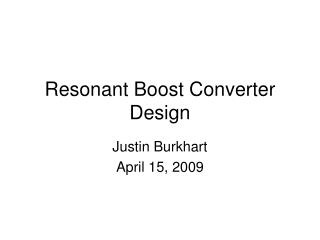DownloadDownload PresentationResonant Boost Converter Design

Resonant Boost Converter Design

Download PresentationResonant Boost Converter Design

- - - - - - - - - - - - - - - - - - - - - - - - - - - E N D - - - - - - - - - - - - - - - - - - - - - - - - - - -
Presentation Transcript

1. Resonant Boost Converter Design Justin Burkhart April 15, 2009

2. Presentation Outline • Project Goals • The Class E Inverter • Resonant Rectifier • LDMOS Device Model • Completed Boost Converter Design

3. Project Goals • Design a resonant boost converter using TI LBC5 process LDMOS devices • Targeted at automotive applications with: • 11-16 Vdc Input • 30 Vdc Output • 10-20 Watts • Highest possible switching frequency with efficiency >80% (if possible)

4. The Class E Inverter Adjusted to deliver DC and AC power to the load • L1 is large choke with only DC current • Switch is opened and closed periodically • Assumed high enough Q such that io is sinusoidal • When the switch opens, circuit is designed such that the voltage V(t) rings back to zero D*T later thus providing a ZVS opportunity

5. The Class E Inverter Adjusted to deliver DC and AC power to the load • Pros • Zero Voltage Switching • Only one ground referenced switch required • Cons • High peak device voltage • Large choke inductor • Sensitive to load changes • Limited minimum output power when C1 is constrained

6. Resonant Input Inductor To see what happens when the value of L1 is reduced break it into two hypothetical inductors, one that carries only DC current and one that carries only AC current. In this configuration, L1-AC is in parallel with C1 • This results with: • Faster Transient Response • Lower Minimum Output Power • Flexibility in choice of C1

7. Equivalent Load for Class E Inverter The load of the Class E Inverter Circuit is tuned to look inductive and can be modeled by an inductor and resistor This equivalent load provides for a simpler design procedure when the inverter will be used in a DC/DC converter

8. Solve for component Values • Solve for the drain voltage by integrating current in the equivalent C1 • Solve for the fundamental Fourier component of drain voltage • Set drain voltage and slope of drain voltage to zero at switch turn on time • Solve for phase of the voltage and current fundamental components • Solve for circuit component values • Assume DC current in the input • Assume sinusoidal current in load

9. Resonant Rectifier To transform the Class E inverter into a DC/DC boost converter, the output of the inverter must be rectified A resonant rectifier is used since the losses incurred by a hard switched rectifier at high frequency are too high to maintain good efficiency The rectifier load is modeled as a constant voltage source since the complete DC/DC converter will use feedback to hold the output voltage constant

10. Resonant Rectifier To maintain ZVS the rectifier must present the same impedance to the Class E Inverter as the L-R load network When designing the rectifier, the goal is to choose L and C such that rectifier will have the same current Io(t) as the L-R circuit. This will maintain ZVS in the inverter.

11. Rectifier Operation Vdiode(t) • Diode turns on when Vdiode(t) goes > Vout • Diode turns off when Io(t) goes < 0 • Initial conditions are known, thus equations for Io(t) and Vdiode(t) can be derived • Using initial conditions, ton and toff must be solved for • Closed form solution is not easily arrived at since equations are non-linear and very messy

12. Rectifier Equivalent Model Rectifier Model 1 Model 2 Total Current: Solid Lines DC Current: Dashed Lines

13. Example Of Rectifier Tuning • Goal: • Adjust the resonant frequency of the rectifier until the input current has the desired phase • Adjust the characteristic impedance of the rectifier until the desired output power is reached Increase Fo Decrease Zo

14. LDMOS Device Model Typical LDMOS Device Model Simplified LDMOS Device Model Parasitic capacitance measurement procedure

15. Device Measurement Data Rds-on Data • Test Device only has 1 bond wire per device terminal • This is a limiting factor in measuring small parasitic device resistances • TI process engineers report that Rds-on for devices in this lot were measured at 165mOhm • This would result with an estimate of about 475mOhm bond wire resistance

16. Device Measurement Results Coss Data Ciss Data 1.45 pF extra parasitic capacitance from measurement 2.3 pF extra parasitic capacitance from measurement

17. Simulated DC/DC Converter

18. Converter Efficiency Efficiency and Output Power Loss Breakdown by Component Gate Drive is Not Included .PARAM Vdc=12 .PARAM F=75e6 .PARAM Vout = 30 .PARAM numCycles=200 .PARAM numCyclesDisp=5 .PARAM ratio = 1.15 .PARAM wo=2*pi*F*ratio .PARAM zo=30 .PARAM Lrect = zo/wo .PARAM Crect = 1/(zo*wo) .PARAM Lpar = .2n .PARAM INDQ = 120

19. Future Work • Device optimization • Parametric variance analysis • Gate driver • Power section prototype • Integrated controller design • Complete converter